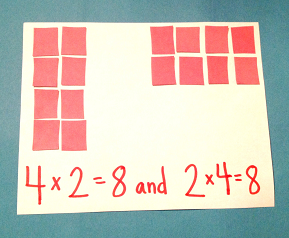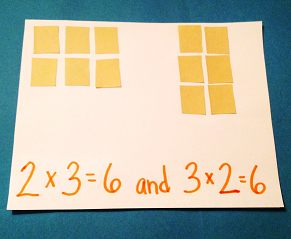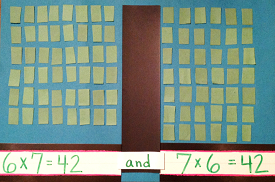# Learning Multiplication Facts to 10 Using Commutative Property

Instructor: Audrey Akins

Audrey has more than a decade of experience teaching elementary. She has a bachelor's in journalism and a master's in education.

Maybe you've heard that learning to multiply requires a really good memory. But actually, once you know some multiplication facts, you can easily solve others. In this lesson, you'll learn how the commutative property is a shortcut to make learning multiplication easier.

## What is the Commutative Property?Imagine that you and a friend are playing a game of memory with multiplication facts. Some cards have the answers and some don't. You turn over a card with the problem 3 x 6 = 18. Then you turn over another card that has 6 x 3 = ___.

Even though you haven't learned your 6 times tables, your friend says you know the answer, thanks to the commutative property. The commutative property is the ability to solve a multiplication problem and get the same answer no matter what order you multiply the numbers in.

Basically, if 3 x 6 = 18, then 6 x 3 = 18, too.

## Learning the 0 to 5 Multiplication FactsKnowing the commutative property is a helpful shortcut for learning the multiplication facts. When you learn one set of multiplication facts, you automatically know the answer when the same fact appears again, but with the numbers flipped around.

Let's look at this more closely. You and your brother are making Easter baskets with eggs stuffed with candy. Your brother fills 2 eggs with 3 pieces of candy. As a multiplication number sentence that's 2 x 3 = 6 candies. You fill 3 eggs with 2 pieces of candy. Your multiplication problem is 3 x 2 = 6 candies.

See how you got the same answer both times? This is because the numbers you were multiplying with (2 and 3) stayed the same - they just moved around. And even though you grouped the candies differently, 3 candies into 2 eggs, and then 2 candies into 3 eggs, you were always working with 6 candies.

As I said earlier, the commutative property is a shortcut for learning multiplication facts. This is because when you learn the multiplication facts for 2, you learn everything from 2 x 0 = 0 to 2 x 10 = 20. Then, when it's time to learn another set of multiplication facts, you already know the answer to any number x 2, because of the commutative property.

So, later on, when you're learning the multiplication facts for 3, you already know 3 x 2 = 6. That's one less fact for you to learn.

## Learning the 6 to 10 Multiplication FactsTo unlock this lesson you must be a Study.com Member.

### Register to view this lesson

Are you a student or a teacher?

Back

### Resources created by teachers for teachers

Over 30,000 video lessons & teaching resources‐all in one place.Video lessonsQuizzes & WorksheetsClassroom IntegrationLesson Plans

I would definitely recommend Study.com to my colleagues. It’s like a teacher waved a magic wand and did the work for me. I feel like it’s a lifeline.

Jennifer B.
TeacherCreate an account to start this course today
Used by over 30 million students worldwide